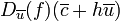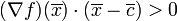# First derivative test for a function of multiple variables

(diff) ← Older revision | Latest revision (diff) | Newer revision → (diff)
This article describes an analogue for functions of multiple variables of the following term/fact/notion for functions of one variable: first derivative test
This article describes a test that can be used to determine whether a point in the domain of a function gives a point of local, endpoint, or absolute (global) maximum or minimum of the function, and/or to narrow down the possibilities for points where such maxima or minima occur.
View a complete list of such tests

## Statement

### What the test is for

The goal of the test is to determine whether a critical point for a function of multiple variables is a point where the function attains a local extreme value, and if so, whether we get a local maximum value or local minimum value.

### What the test says: one-sided directional version

Suppose$f$ is a function of a vector variable and$\overline{c}$ is a point in the domain of$f$. Suppose$\overline{u}$ is any unit vector. Then, we have the following:

Continuity and differentiability assumptions Hypothesis on sign of directional derivative Conclusion for$f$ at$\overline{c}$ along the outward$\overline{u}$-direction
The function$h \mapsto f(\overline{c} + h\overline{u})$ is right continuous at$h = 0$ and differentiable for$h$ to the immediate right of zero. In other words, the directional derivative along the direction of$\overline{u}$ exists on the outward half-line from$\overline{c}$ along$\overline{u}$. The directional derivative$D_{\overline{u}}(f)(\overline{c} + h\overline{u})$ is positive for$h$ to the immediate right of 0. strict local minimum
The function$h \mapsto f(\overline{c} + h\overline{u})$ is right continuous at$h = 0$ and differentiable for$h$ to the immediate right of zero. In other words, the directional derivative along the direction of$\overline{u}$ exists on the outward half-line from$\overline{c}$ along$\overline{u}$. The directional derivative$D_{\overline{u}}(f)(\overline{c} + h\overline{u})$ is negative for$h$ to the immediate right of 0. strict local maximum

### What the test says: linear directions version

Suppose$f$ is a function of a vector variable and$\overline{c}$ is a point in the domain of$f$. Then, we have the following:

Continuity and differentiability assumptions Hypothesis on sign of directional derivative Conclusion for$f$ at$\overline{c}$$f$ is continuous in every linear direction at$\overline{c}$ and along every linear direction, the directional derivative of$f$ in that direction exists close to$\overline{c}$ For every unit vector$\overline{u}$, the directional derivative$D_{\overline{u}}(f)(\overline{c} + h\overline{u})$ is positive for$h$ to the immediate right of 0. strict local minimum$f$ is continuous in every linear direction at$\overline{c}$ and along every linear direction, the directional derivative of$f$ in that direction exists close to$\overline{c}$ For every unit vector$\overline{u}$, the directional derivative$D_{\overline{u}}(f)(\overline{c} + h\overline{u})$ is negative for$h$ to the immediate right of 0. strict local maximum

### What the test says: continuous and differentiable version

This version is weaker than the linear directions version, because it is possible to construct situations where the linear directions version is conclusive but the "continuous and differentiable" version isn't. However, in most practical situations, the two tests have the same power and same meaning.

Continuity and differentiability assumptions Hypothesis on dot product of gradient vector and difference vector Conclusion for$f$ at$\overline{c}$$f$ is continuous at$\overline{c}$ and differentiable in an open ball containing$\overline{c}$, except possibly at$\overline{c}$. Here, differentiable means that the gradient vector exists. For$\overline{x}$ sufficiently close to$\overline{c}$,$(\nabla f)(\overline{x}) \cdot (\overline{x} - \overline{c}) > 0$. strict local minimum$f$ is continuous at$\overline{c}$ and differentiable in an open ball containing$\overline{c}$, except possibly at$\overline{c}$. Here, differentiable means that the gradient vector exists. For$\overline{x}$ sufficiently close to$\overline{c}$,$(\nabla f)(\overline{x}) \cdot (\overline{x} - \overline{c}) < 0$. strict local maximum